# Averaging example

New for May 2020. Averaging is used in measurements and sensing in order to improve data.  Here we will provide a non-microwave example that shows how to improve accuracy of data by gathering multiple measurements, each of limited accuracy, and averaging them. Here's the premise:

Suppose every day on your way home from work you pass two landmarks that you want to measure the exact distance between, using your car's odometer. This is in the days before GPS, so there is no shortcut. And your odometer does not have tenths of a mile (or kilometers), just whole numbers.

Further suppose that the points of interest are exactly 2pi miles (or kilometers) apart. So, 6.283.xxxx miles. When you look at your odometer at the two landmarks and subtract the numbers, it usually indicates they are 6 miles apart, but sometimes 7 miles. Let's model that in Excel using a random number generator and see what happens to the average distance after many trips.

Below is a snapshot of the data in our spreadsheet (if you want a copy, just ask)... we averaged 10,000 measurements, you are looking at just the first 25. Let's point out a few things: "Pi" in Excel only has 15 digits, and so does a random number. In the image below,  the first column is the index of the measurement.  The next column is a random number between 0 and 1.   After that is an odometer start number, which is not really relevant, but it goes with the story. Then, the actual mileage start adds or subtracts the random number depending on if it is more or less than 0.5 (we assumed that that the odometer "flips" at the half-way point). Then the actual end mileage is determined by adding the exact distance of 2pi. Next the actual end mileage is rounded to a whole number and reported by the odometer. Odometer distance is calculated by subtracting odometer readings and of either 6 or 7. Then a running average is computed, which can be compared to the true distance of 2pi. The last column is the error in percent, which we expect to go on a downward trend.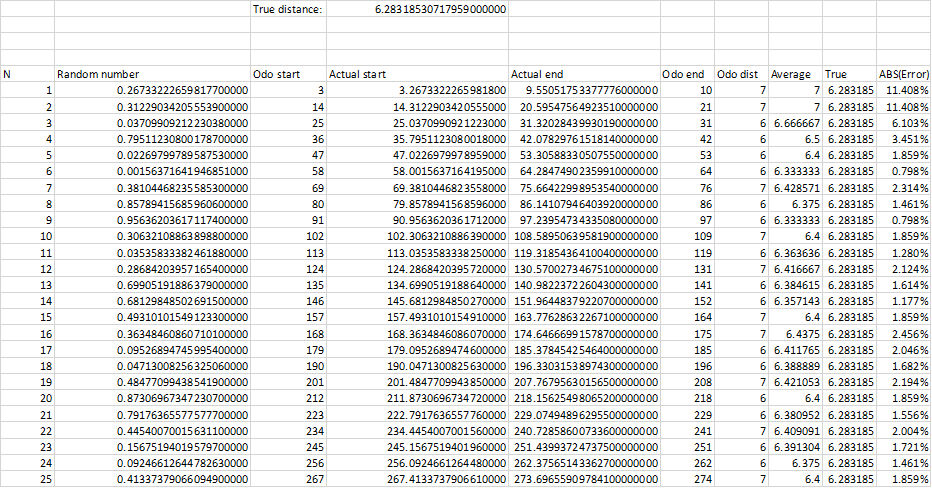The random number regenerates every time you make a change or store the file, and of course there is no way to go backwards and look at previous trials.  We plotted three cases, showing measured and true mileage on one plot (log scale on number of trials) and percent effort (log-log scale).  Here's Case 1: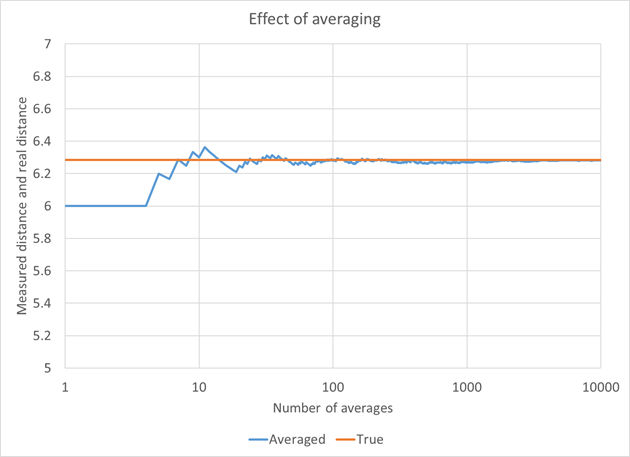Here is the percent error for Case 1. Note that this is a log-log plot. Let's further quantify the errors so you can appreciate how small they are trending towards, compared to the maximum possible error of 11.4% (measure 7 for distance of 2pi)

11.4% is 3782 feet
0.1% is 33 feet
0.01% is 3.3 feet

Or if you are are on the metric system,

11.4% is 716 meters
0.1% is 6.3 meters
0.01% is 630mm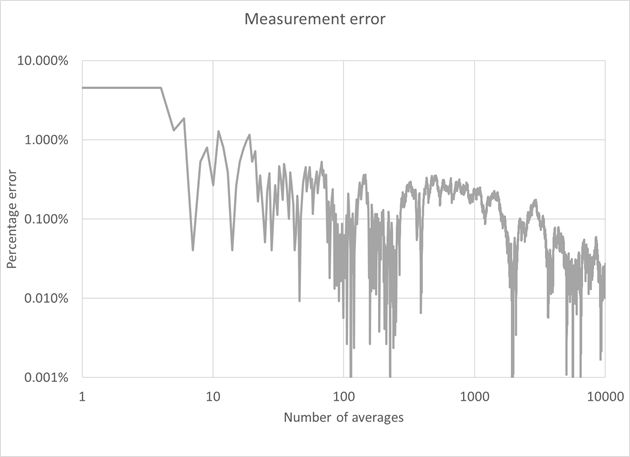Here is Case 2: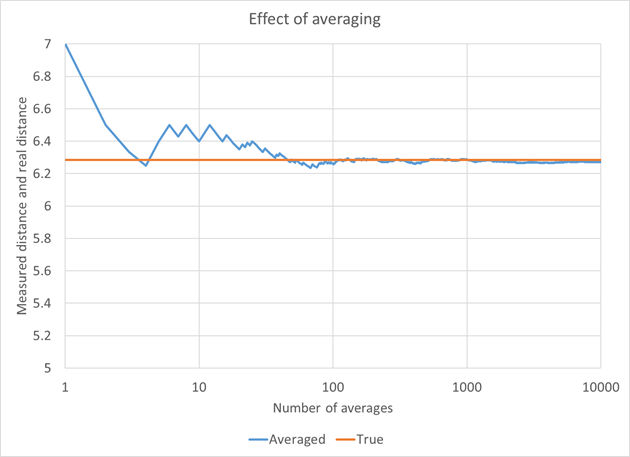Here is the percent error for Case 2.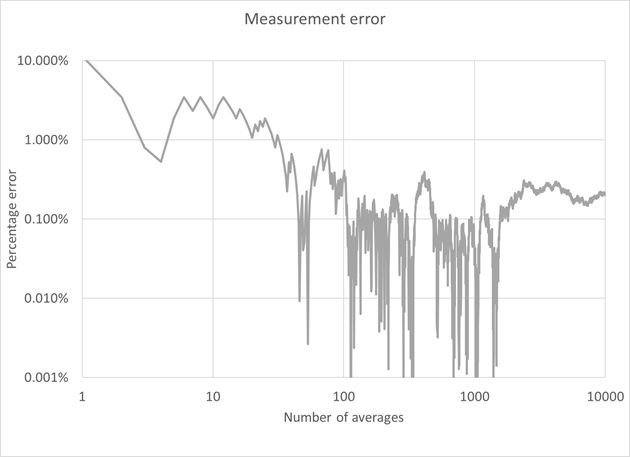Here is Case 3: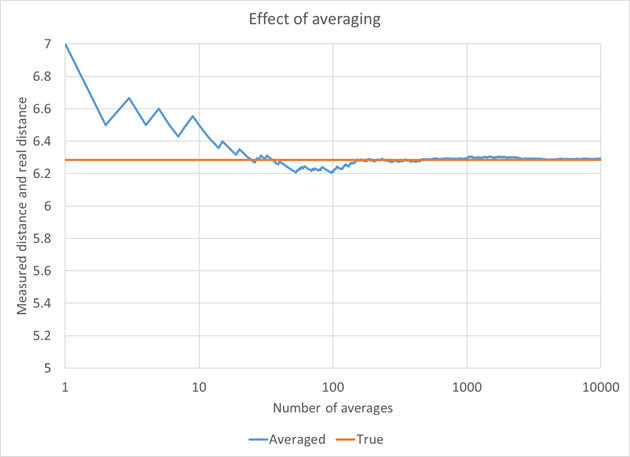And here is the percent error for Case 3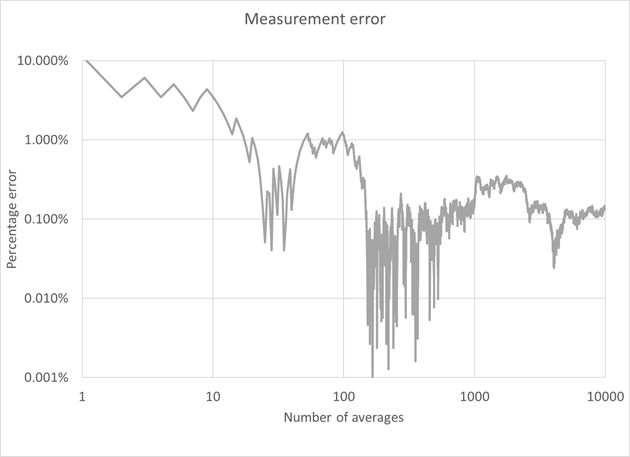As expected, the error has a general downward trend with the number of averages. But sometimes the errors after thousands of cases are greater than the errors after 100s of cases.  Excel is not a good platform to try to run 100,000 or 1,000,000 cases, maybe the error would reduce further.  Or maybe the Excel random number generator is not really that random?

It looks like we can say that we consistently have an error below 1% for 1000 averages and up.

Author : Unknown Editor